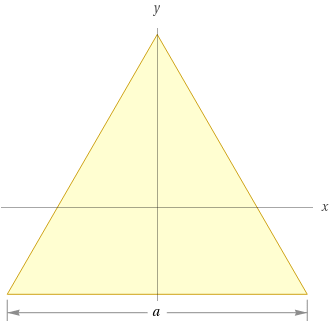Search IntMath
Close

# Exploring the Height of an Equilateral Triangle in Geometry## What is an Equilateral Triangle?

An equilateral triangle is a type of triangle with three sides of equal length and three angles that are all the same measure. It is one of the most basic shapes in geometry, and its understanding and use is essential to the study of more complicated geometric shapes and concepts. Because the sides of an equilateral triangle are all equal, it is also known as an equilateral.

An equilateral triangle has three sides of equal length, and each angle is 60�. You can calculate the length of each side by taking the square root of 3, multiplied by the length of one side. For example, if the side of the equilateral triangle is 2, the length of each side would be 2v3 or approximately 3.46.

## How to Calculate the Height of an Equilateral Triangle

The height of an equilateral triangle is the perpendicular distance from the triangle's base to its highest point. This height can be calculated by taking the length of one side and multiplying it by the square root of 3, divided by 2. For example, if the side of the equilateral triangle is 2, the height of the triangle would be 2v3/2 or approximately 1.73.

In addition to calculating the height of an equilateral triangle, you can also calculate the triangle's perimeter and area. The perimeter of an equilateral triangle is equal to the sum of the lengths of all three sides. For example, if the side of the triangle is 2, the perimeter of the triangle would be 6. The area of an equilateral triangle can be calculated by taking one-half of the product of the length of the side and the height of the triangle. In this case, the area of the triangle would be 2*1.73, or 3.46.

## Practice Problems

Now that you know how to calculate the height of an equilateral triangle, let's practice! Here are some practice problems to get you started.

1. What is the height of an equilateral triangle with a side length of 5?
2. What is the perimeter of an equilateral triangle with a side length of 4?
3. What is the area of an equilateral triangle with a side length of 3?
4. What is the length of one side of an equilateral triangle with a height of 2?
5. What is the perimeter of an equilateral triangle with an area of 24?

• The height of an equilateral triangle with a side length of 5 is 8.66.
• The perimeter of an equilateral triangle with a side length of 4 is 12.
• The area of an equilateral triangle with a side length of 3 is 4.24.
• The length of one side of an equilateral triangle with a height of 2 is 3.46.
• The perimeter of an equilateral triangle with an area of 24 is 24.

## Summary

In this article, we explored the height of an equilateral triangle in geometry. We learned that an equilateral triangle has three sides of equal length and three angles that are all the same measure. We discussed how to calculate the height, perimeter, and area of an equilateral triangle. Finally, we practiced our skills with some practice problems. Now you should have a better understanding of the height of an equilateral triangle in geometry.

## FAQ

### What is height of equilateral triangle?

The height of an equilateral triangle is the length of a line segment from the midpoint of one side of the triangle to the opposite vertex.

### How do you derive the height of an equilateral triangle?

To derive the height of an equilateral triangle, you can use the Pythagorean theorem or the trigonometric ratios of sine, cosine, and tangent.

### How do you find the height of a triangle in geometry?

To find the height of a triangle in geometry, you can use the Pythagorean theorem or the trigonometric ratios of sine, cosine, and tangent.

### What is the area and height of equilateral triangle?

The area of an equilateral triangle is equal to the length of one side squared multiplied by the square root of 3, divided by 4. The height of an equilateral triangle is the length of a line segment from the midpoint of one side of the triangle to the opposite vertex.

## Problem SolverThis tool combines the power of mathematical computation engine that excels at solving mathematical formulas with the power of GPT large language models to parse and generate natural language. This creates math problem solver thats more accurate than ChatGPT, more flexible than a calculator, and faster answers than a human tutor. Learn More.